# numpy.polynomial.hermite.hermgauss¶

numpy.polynomial.hermite.hermgauss(deg)[source]

Computes the sample points and weights for Gauss-Hermite quadrature. These sample points and weights will correctly integrate polynomials of degree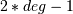or less over the interval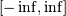with the weight function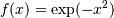.

Parameters: deg : int Number of sample points and weights. It must be >= 1. x : ndarray 1-D ndarray containing the sample points. y : ndarray 1-D ndarray containing the weights.

Notes

The results have only been tested up to degree 100, higher degrees may be problematic. The weights are determined by using the fact that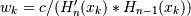whereis a constant independent of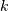and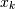is the k’th root of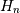, and then scaling the results to get the right value when integrating 1.

#### Previous topic

numpy.polynomial.hermite.hermpow

#### Next topic

numpy.polynomial.hermite.hermweight